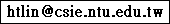Hsuan-Tien Lin

Home | MOOCs | AIsk | Courses | Research Group | Awards | Publications | Presentations | Programs/Data

AIsk (Ask about Artificial Intelligence) is a special project of mine to share some knowledge of AI to the Mandarin-speaking society from the popular science perspective. The knowledge will be presented in the form of short videos. For those interested in some deeper contents, you can check the MOOCs that I offered and the textbook Learning from Data: A Short Course that I co-authored.

The slides of AIsk below are available as is with no explicit or implied warranties. The slides themselves are shared by CC-BY-NC 3.0, but the copyright of all materials (figures in particular) remain with the original copyright holder..

Here are some quick links for each MOOC. The erratas are here.

 Machine Learning FoundationsMOOC all handout slides free youtube videos Machine Learning TechniquesMOOC all handout slides free youtube videos

Detailed outlines for each MOOC, along with the presentation sldies, are listed below.

Machine Learning Foundations

 When can machines learn? Lecture 1 the learning problem: Course Introduction What is Machine Learning Applications of Machine Learning Components of Machine Learning Machine Learning and Other Fields handout slides; presentation slides Lecture 2 learning to answer yes/no: Perceptron Hypothesis Set Perceptron Learning Algorithm (PLA) Guarantee of PLA Non-Separable Data handout slides; presentation slides Lecture 3 types of learning: Learning with Different Output Space Learning with Different Data Label Learning with Different Protocol Learning with Different Input Space handout slides; presentation slides Lecture 4 feasibility of learning: Learning is Impossible? Probability to the Rescue Connection to Learning Connection to Real Learning handout slides; presentation slides Why can machines learn? Lecture 5 training versus testing: Recap and Preview Effective Number of Lines Effective Number of Hypotheses Break Point handout slides; presentation slides Lecture 6 theory of generalization: Restriction of Break Point Bounding Function: Basic Cases Bounding Function: Inductive Cases A Pictorial Proof handout slides; presentation slides Lecture 7 the VC dimension: Definition of VC Dimension VC Dimension of Perceptrons Physical Intuition of VC Dimension Interpreting VC Dimension handout slides; presentation slides Lecture 8 noise and error: Noise and Probabilistic Target Error Measure Algorithmic Error Measure Weighted Classification handout slides; presentation slides How can machines learn? Lecture 9 linear regression: Linear Regression Problem Linear Regression Algorithm Generalization Issue Linear Regression for Binary Classification handout slides; presentation slides Lecture 10 logistic regression: Logistic Regression Problem Logistic Regression Error Gradient of Logistic Regression Error Gradient Descent handout slides; presentation slides Lecture 11 linear models for classification: Linear Models for Binary Classification Stochastic Gradient Descent Multiclass via Logistic Regression Multiclass via Binary Classification handout slides; presentation slides Lecture 12 nonlinear transformation: Quadratic Hypotheses Nonlinear Transform Price of Nonlinear Transform Structured Hypothesis Sets handout slides; presentation slides How can machines learn better? Lecture 13 hazard of overfitting: What is Overfitting? The Role of Noise and Data Size Deterministic Noise Dealing with Overfitting handout slides; presentation slides Lecture 14 regularization: Regularized Hypothesis Set Weight Decay Regularization Regularization and VC Theory General Regularizers handout slides; presentation slides Lecture 15 validation: Model Selection Problem Validation Leave-One-Out Cross Validation V-Fold Cross Validation handout slides; presentation slides Lecture 16 three learning principles: Occam's Razor Sampling Bias Data Snooping Power of Three handout slides; presentation slides

Machine Learning Techniques

 embedding numerous features Lecture 1 linear support vector machine: Course Introduction Large-Margin Separating Hyperplane Standard Large-Margin Problem Support Vector Machine Reasons behind Large-Margin Hyperplane handout slides; presentation slides Lecture 2 dual support vector machine: Motivation of Dual SVM Lagrange Dual SVM Solving Dual SVM Messages behind Dual SVM handout slides; presentation slides Lecture 3 kernel support vector machine: Kernel Trick Polynomial Kernel Gaussian Kernel Comparison of Kernels handout slides; presentation slides Lecture 4 soft-margin support vector machine: Motivation and Primal Problem Dual Problem Messages behind Soft-Margin SVM Model Selection handout slides; presentation slides Lecture 5 kernel logistic regression: Soft-Margin SVM as Regularized Model SVM versus Logistic Regression SVM for Soft Binary Classification Kernel Logistic Regression handout slides; presentation slides Lecture 6 support vector regression: Kernel Ridge Regression Support Vector Regression Primal Support Vector Regression Dual Summary of Kernel Models handout slides; presentation slides combining predictive features Lecture 7 blending and bagging: Motivation of Aggregation Uniform Blending Linear and Any Blending Bagging (Bootstrap Aggregation) handout slides; presentation slides Lecture 8 adaptive boosting: Motivation of Boosting Diversity by Re-weighting Adaptive Boosting Algorithm Adaptive Boosting in Action handout slides; presentation slides Lecture 9 decision tree: Decision Tree Hypothesis Decision Tree Algorithm Decision Tree Heuristics in C&RT Decision Tree in Action handout slides; presentation slides Lecture 10 random forest: Random Forest Algorithm Out-Of-Bag Estimate Feature Selection Random Forest in Action handout slides; presentation slides Lecture 11 gradient boosted decision tree: Adaptive Boosted Decision Tree Optimization View of AdaBoost Gradient Boosting Summary of Aggregation Models handout slides; presentation slides distilling hidden features Lecture 12 neural network: Motivation Neural Network Hypothesis Neural Network Learning Optimization and Regularization handout slides; presentation slides Lecture 13 deep learning: Deep Neural Network Autoencoder Denoising Autoencoder Principal Component Analysis handout slides; presentation slides Lecture 14 radial basis function network: RBF Network Hypothesis RBF Network Learning k-Means Algorithm k-Means and RBF Network in Action handout slides; presentation slides Lecture 15 matrix factorization: Linear Network Hypothesis Basic Matrix Factorization Stochastic Gradient Descent Summary of Extraction Models handout slides; presentation slides happy learning! Lecture 16 finale: Feature Exploitation Techniques Error Optimization Techniques Overfitting Elimination Techniques Machine Learning in Practice handout slides; presentation slides

 Last updated at CST 11:31, August 02, 2021 Please feel free to contact me: